# Mutual-Positive-Feedback Mathematics

L. David Roper

http://www.roperld.com/personal/roperldavid.htm
6 April, 2016

## Introduction

There are many physical situations where two or more variables mutually interact to create positive feedback. An example is Earth average temperature and carbon-dioxide concentration in the atmosphere.

This article devises a simple model to account for positive feedback between two variables.

Consider two variables, A and B, that mutually interact to create positive feedback. Assume that the feedback time dependence is governed by the hyperbolic tangent function, tanh (x). (See http://www.roperld.com/science/hyperbolictangentworld.pdf). That is, there is a time delay for one variable to react to the change in the other variable in the same direction; the time delay may be different for each of the two variables.

The following form will be used to incorporate the hyperbolic tangent function in the feedback mechanism: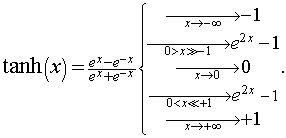Its graph is as follows: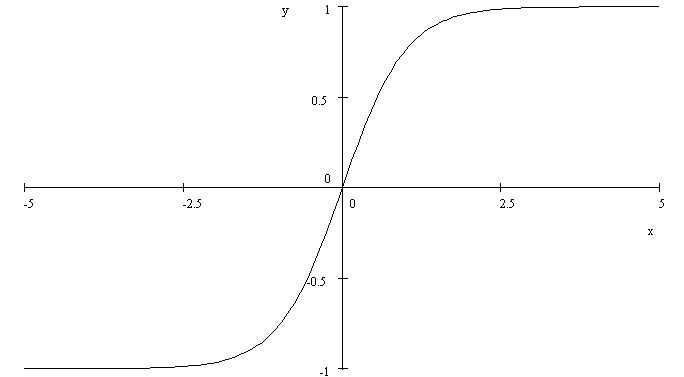A more general function is needed for real-world fits to natural phenomena, as given in the following equation:.

For example,is: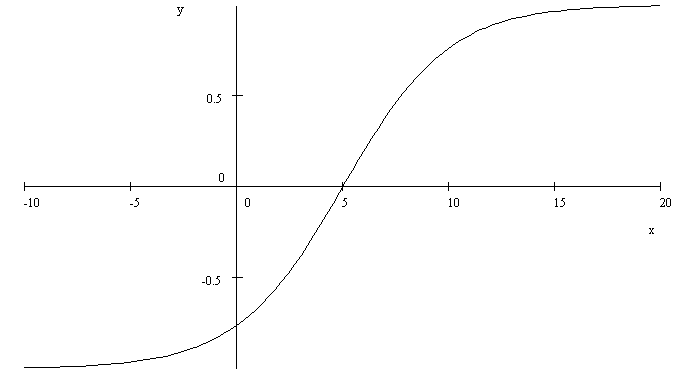Sometimes the hyperbolic tangent is needed to start from 0 instead of -1 (zero-start hyperbolic tangent):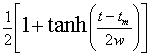.

## Model for Mutual-Positive Feedback between Two Variables

The model used here for mutual-positive feedback between two variables is an iterative one that converges very rapidly.

For the simplest realistic case, consider that variable A changes slowly from some steady-state value to a new steady-state value, arbitrarily set to zero, by the hyperbolic tangent from its midpoint (value 0). Then assume that, to a first-order approximation, variable B changes gradually from arbitrary value zero to some new value by the zero-start hyperbolic tangent. To a first-order approximation:Similarly, when B changes as above in first order, A then changes gradually by the zero-start hyperbolic tangent to some new value. So, the second-order approximation is: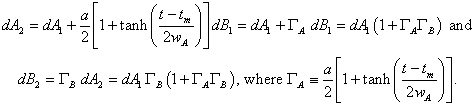The third-order approximation is: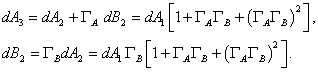The fourth-order approximation is:The nth-order approximation is: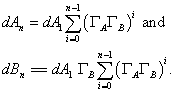The result would be whenHowever, the series may converge for just a few terms because of the asymptotic behavior of the hyperbolic tangent.

## Example of the Model

Consider the simple case in which the mutual interaction is symmetrical:That is, the time delay is the same for B when A changes as it is for A when B changes (parameters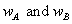) and the amount of change is the same for both A and B (parameters).

The first four approximations are show in this graph: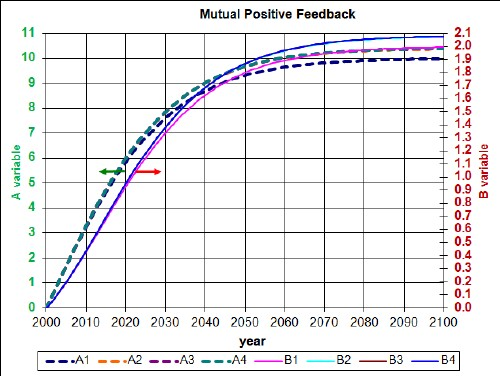For this symmetric model highly accurate convergence occurs at the 3rd approximation and the result is quite good in the 2nd approximation. Even the 1st approximation is a good estimate for variable B.

A correlation calculation for the 4th approximation gives the correlations: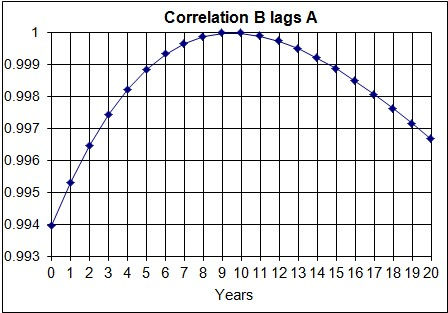So, B lags A by about 9.5 years, because A was changed first. If B were changed first, A would lag B by the same number of years for a symmetrical mutual interaction. Therefore, two variables that mutually interact symmetrically to give mutual-positive feedback will lead or lag each other by the same number of time units depending on which one is changed first after a steady state situation.

## Mutual-Positive-Feedback Model for Three Variables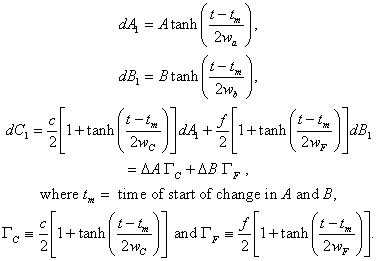The second-order approximation is: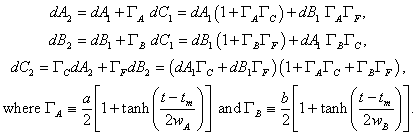The third-order approximation is: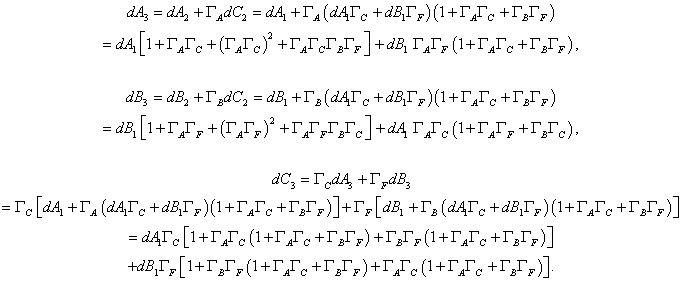## Conclusion

The mutual-positive-feedback model used in this work shows that which of two such interacting variables leads the other depends on which one was changed by interaction with some other variable.